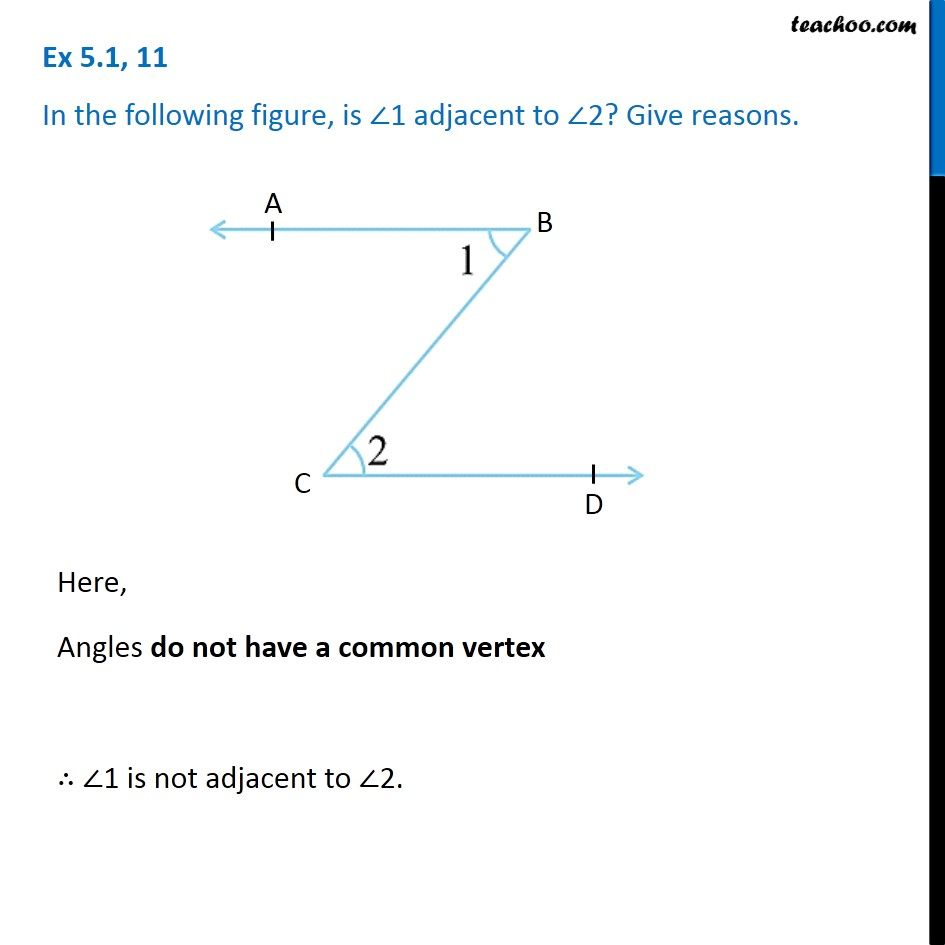1. Chapter 5 Class 7 Lines and Angles
2. Concept wise
3. Adjacent Angles, Linear Pair of angles, Vertically Opposite angles

Transcript

Ex 5.1, 11 Chapter 5 Class 7 Lines and Angles (of the NCERT Book) In the following figure, is ∠1 adjacent to ∠2? Give reasons. Here, Angles do not have a common vertex ∠1 has vertex as point B ∠2 has vertex as point C ∴ ∠1 is not adjacent to ∠2.

Adjacent Angles, Linear Pair of angles, Vertically Opposite angles